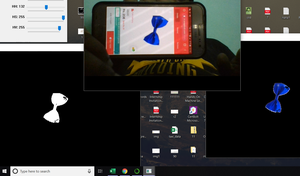# Detecting objects of similar color in Python using OpenCV

• Difficulty Level : Expert
• Last Updated : 23 Jun, 2021

OpenCV is a library of programming functions mainly aimed at real-time computer vision.

In this article, we will see how to get the objects of the same color in an image. We can select a color by slide bar which is created by the cv2 command cv2.createTrackbar.

#### Libraries needed:

```OpenCV
Numpy```

#### Approach:

First of all, we need to read the image which is in our local folder using cv2.imread( ). For filtering a specific color we need to convert image into HSV format which is hue, saturation, and value and mask the image using cv2.inRange( ) by providing lower and upper bounds of RGB values we wanted to filter which gives us a black and white image where the images with the color of our interests are in white and remaining are in black. we can get back the images with the specified color which we gave it by trackbar by doing cv2 bitwise_and operation.

Code:

## Python3

 `# import required library``import` `cv2``import` `numpy as np``import` `matplotlib.pyplot as plt`` ` `# create a video object``# for capture the frames.``# for Webcamera we pass 0 ``# as an argument ``cap ``=` `cv2.VideoCapture(``0``)`` ` `# define a empty function ``def` `nothing(x):``    ``pass`` ` `# set windown name``cv2.namedWindow(``'Tracking'``)`` ` `# Creates a trackbar and attaches ``# it to the specified window ``# with nothing function``cv2.createTrackbar(``"LH"``, ``"Tracking"``,``                   ``0``, ``255``, nothing)``cv2.createTrackbar(``"LS"``, ``"Tracking"``, ``                   ``0``, ``255``, nothing)``cv2.createTrackbar(``"LV"``, ``"Tracking"``, ``                   ``0``, ``255``, nothing)``cv2.createTrackbar(``"HH"``, ``"Tracking"``, ``                   ``0``, ``255``, nothing)``cv2.createTrackbar(``"HS"``, ``"Tracking"``, ``                   ``0``, ``255``, nothing)``cv2.createTrackbar(``"HV"``, ``"Tracking"``,``                   ``0``, ``255``, nothing)`` ` `# This drives the program ``# into an infinite loop. ``while` `True``:``   ` `    ``# Captures the live stream frame-by-frame``    ``_, frame ``=` `cap.read()``     ` `    ``# Converts images from BGR to HSV ``    ``hsv ``=` `cv2.cvtColor(frame, ``                       ``cv2.COLOR_BGR2HSV)``     ` `    ``# find LH trackbar position``    ``l_h ``=` `cv2.getTrackbarPos(``"LH"``,``                             ``"Tracking"``)``    ``# find LS trackbar position``    ``l_s ``=` `cv2.getTrackbarPos(``"LS"``,``                             ``"Tracking"``)``    ``# find LV trackbar position``    ``l_v ``=` `cv2.getTrackbarPos(``"LV"``, ``                             ``"Tracking"``)``    ``# find HH trackbar position``    ``h_h ``=` `cv2.getTrackbarPos(``"HH"``, ``                             ``"Tracking"``)``    ``# find HS trackbar position``    ``h_s ``=` `cv2.getTrackbarPos(``"HS"``,``                             ``"Tracking"``)``    ``# find HV trackbar position``    ``h_v ``=` `cv2.getTrackbarPos(``"HV"``,``                             ``"Tracking"``)``    ``# create a given numpy array``    ``l_b ``=` `np.array([l_h, l_s,``                    ``l_v])``    ``# create a given numpy array``    ``u_b ``=` `np.array([h_h, h_s,``                    ``h_v])``    ``# create a mask``    ``mask ``=` `cv2.inRange(hsv, l_b,``                       ``u_b)``    ``# applying bitwise_and operation``    ``res ``=` `cv2.bitwise_and(frame, ``                          ``frame, mask ``=` `mask)``     ` `    ``# display frame, mask``    ``# and res window``    ``cv2.imshow(``'frame'``, frame)``    ``cv2.imshow(``'mask'``, mask)``    ``cv2.imshow(``'res'``, res)``     ` `    ``# wait for 1 sec``    ``k ``=`  `cv2.waitKey(``1``)``     ` `    ``# break out of while loop``    ``# if k value is 27``    ``if` `k ``=``=` `27``:``        ``break``         ` `# release the captured frames ``cap.release()`` ` `# Destroys all windows. ``cv2.destroyAllWindows()`

Output:My Personal Notes arrow_drop_up